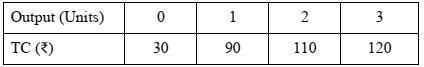# ISC Class 12 Economics 2019 Question Paper

Answer Question 1 (compulsory) from Part I and five questions from Part II.

### Part I (20 Marks)

Question 1

Answer briefly each of the following questions (i) to (x): [10×2]

(i) What is meant by product differentiation in monopolistic competition?

(ii) Explain an indifference map, with the help of a diagram.

(iii) Give two examples of each of the following:

1. Revenue receipts of the government.
2. Revenue expenditure of the government.

(iv) With the help of a diagram, state the behaviour of MP when:

1. TP of the variable factor reaches maximum.
2. TP of the variable factor falls.

(v) What is meant by High Powered Money?

(vi) Distinguish between depreciation and devaluation.

(vii) Explain any two precautions to be taken while calculating national income by income method.

(viii) Differentiate between accounting cost and opportunity cost.

(ix) With the help of diagrams, show when the elasticity of supply is:

1. greater than one.
2. equal to one.

(x) What is meant by investment multiplier?

### Part II (60 Marks)

Question 2

(a) How does an increase in income affect the demand for the following: 

1. A normal good
2. An inferior good

(b) Discuss any three reasons for the leftward shift of a supply curve. 

(c) Explain how a consumer attains equilibrium using indifference curve analysis. 

Question 3

(a) Discuss two differences between returns to scale and returns to a variable factor. 

(b) With the help of a diagram, explain the relationship between AR and MR of a firm under imperfect competition. 

(c) Discuss any four features of monopoly market. 

Question 4

(a) Explain the various degrees of price elasticity of demand at different points on a straight line demand curve. 

(b) Show with the help of a diagram, how a perfectly competitive firm earns normal profit in short run equilibrium. 

(c) Explain with the help of diagrams how equilibrium price changes when there is simultaneous increase of both, demand and supply. 

Question 5

(a) Discuss any two exceptions to the law of demand. 

(b) Study the cost function of a firm given below:Calculate:

1. AFC
2. AC
3. MC

(c) A producer is in equilibrium when MR = MC. Explain this statement with the help of a diagram. 

Question 6

(a) Explain how public expenditure can be used as an instrument of fiscal policy to solve the problem of: 

1. Income inequality
2. Inflation

(b) Differentiate between the revenue and capital components of the union budget. 

(c) Discuss briefly the various components of balance of payment. 

Question 7

(a) Discuss any two limitations of credit creation by commercial banks. 

(b) Explain two secondary functions of money. 

(c) Discuss any two qualitative methods and any two quantitative methods of credit control used by the Central Bank. 

Question 8

(a) What is meant by average propensity to consume? Explain its relationship with average propensity to save. 

(b) Discuss any two fiscal measures to correct a situation of deficient demand in an economy. 

(c) Explain how equilibrium level of income can be determined with the help of saving and investment approach. 

Question 9

(a) Draw a well-labelled diagram to show a circular flow of income in a two sector model. What happens to the flow of income when savings equals investment? 

(b) What is meant by economic welfare? Explain how GDP is an indicator of economic welfare. 

(c) From the following data, calculate National Income by Output method and Income method: 

Item : ₹ in crores

1. Value of output : 2500
2. Value of intermediate consumption : 1300
3. Subsidies : 40
4. Rent : 110
5. Employer’s contribution to social security : 30
6. Profit : 50
7. Wages and salaries : 340
8. Interest : 10
9. Mixed income of self-employed : 360
10. Indirect tax : 180
11. NFIA : (-)30
12. Consumption of fixed capital : 160